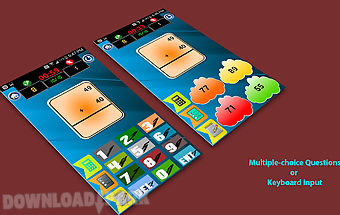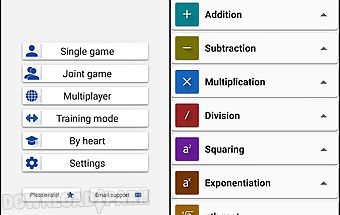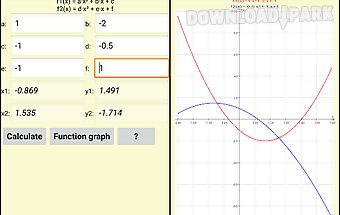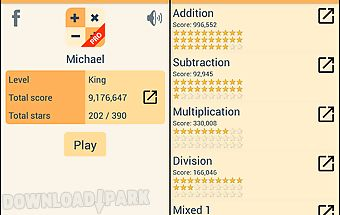# Math games free Android App

### Free Apk Files » Other Apps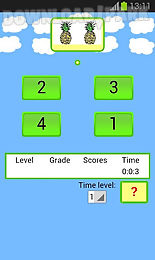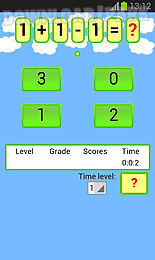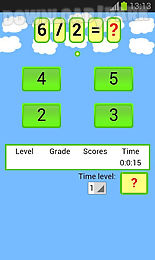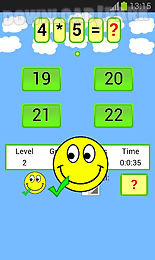The description of Math games free: This app allows you to help children with math skills. Program for kids. Excellent tool for school. Learn counting numbers learn and practice: addition subtraction multiplication division. Four basic math operations. Children learn basic math. How to add and subtract. Mental maths skills. Become good with numbers. Improve your math potential. Exercises times tables. Activities to encourage counting. Kids familiar with numbers. Understanding mathematical concepts. Kidslearn to solve problems . Motivate your children to love math. Fun educational math. Numbers activities for kids. Math games that are easy to make. Exercises games for arithmetic. Interactive activities. An useful way to learn cational games for kids. Become good with numbers. All you have to do is to choose the right answer. For phone and tablet. Features: score. Levels. Level change automatically. Time level. Maths lessons..

## Similar Apps to Math games free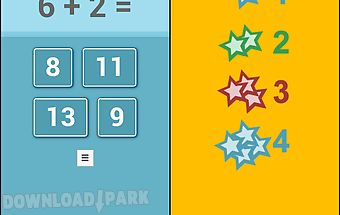Math games for kids
Kids can have fun while learning to solve math exercises with the math games. This app is cool math games for kids all free. This app helps to practice mathematics. Prog..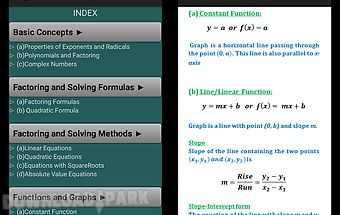Math formulae lite (free)
Math formulae lite is one unique and comprehensive app that is particularly designed as a one stop solution for college grade higher grade students. It lists out all the..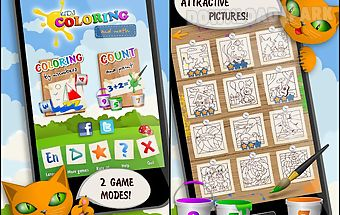Kids coloring and math free
With our unique game your child will solve the elementary math problems with great pleasure! The bright math coloring will allow your child not only to show the creative..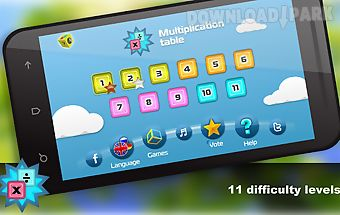Math.multiplication table free
you re certainly going to get your money s wroth out of mental math multiplication table (c) with math multiplication table your child will love math and improve multip..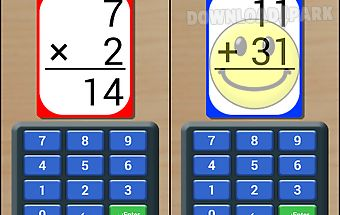Math flash cards (free)
Math flash cards (free):children can build and improve essential math skills in: addition subtraction multiplication and division. Features include: new: option to allow..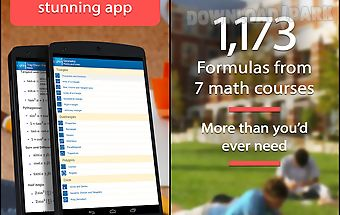Formulae helper free - math
Formulae helper free the best ref handbook app of math formulas on the market. From the creators of math helper! It provides easy access to formulas in algebra trigonome..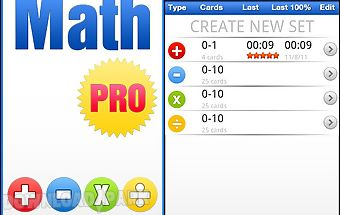Math pro - math game for kids
math pro math game for kids by studios parents and students have asked for a more advance version of our very popular math practice flash cards app and here it is! A fu..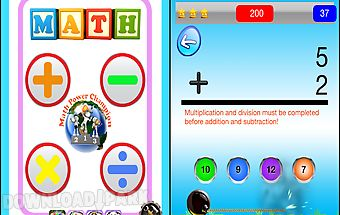Math practice flash cards free
Math practice flash cards for kids by everykidsapps. A fun game to practice addition subtraction multiplication or division. Features: addition subtraction multiplicatio..